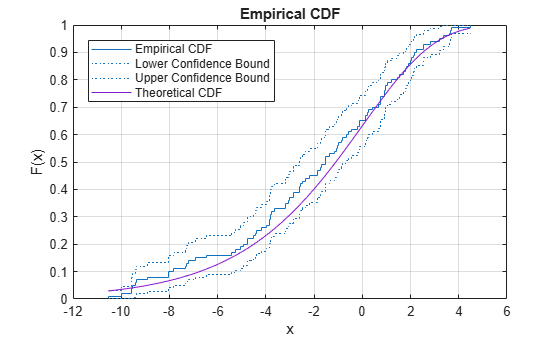Documentation

# cdfplot

Empirical cumulative distribution function (cdf) plot

## Syntax

``cdfplot(x)``
``h = cdfplot(x)``
``[h,stats] = cdfplot(x)``

## Description

example

````cdfplot(x)` creates an empirical cumulative distribution function (cdf) plot for the data in `x`. For a value t in `x`, the empirical cdf F(t) is the proportion of the values in `x` less than or equal to t.```
````h = cdfplot(x)` returns a handle of the empirical cdf plot line object. Use `h` to query or modify properties of the object after you create it. For a list of properties, see Line Properties.```
````[h,stats] = cdfplot(x)` also returns a structure including summary statistics for the data in `x`.```

## Examples

collapse all

Plot the empirical cdf of a sample data set and compare it to the theoretical cdf of the underlying distribution of the sample data set. In practice, a theoretical cdf can be unknown.

Generate a random sample data set from the extreme value distribution with a location parameter of 0 and a scale parameter of 3.

```rng('default') % For reproducibility y = evrnd(0,3,100,1);```

Plot the empirical cdf of the sample data set and the theoretical cdf on the same figure.

```cdfplot(y) hold on x = linspace(min(y),max(y)); plot(x,evcdf(x,0,3)) legend('Empirical CDF','Theoretical CDF','Location','best') hold off```The plot shows the similarity between the empirical cdf and the theoretical cdf.

Alternatively, you can use the `ecdf` function. The `ecdf` function also plots the 95% confidence intervals estimated by using Greenwood's Formula. For details, see Greenwood’s Formula.

```ecdf(y,'Bounds','on') hold on plot(x,evcdf(x,0,3)) grid on title('Empirical CDF') legend('Empirical CDF','Lower Confidence Bound','Upper Confidence Bound','Theoretical CDF','Location','best') hold off```Perform the one-sample Kolmogorov-Smirnov test by using `kstest`. Confirm the test decision by visually comparing the empirical cumulative distribution function (cdf) to the standard normal cdf.

Load the `examgrades` data set. Create a vector containing the first column of the exam grade data.

```load examgrades test1 = grades(:,1);```

Test the null hypothesis that the data comes from a normal distribution with a mean of 75 and a standard deviation of 10. Use these parameters to center and scale each element of the data vector, because `kstest` tests for a standard normal distribution by default.

```x = (test1-75)/10; h = kstest(x)```
```h = logical 0 ```

The returned value of `h = 0` indicates that `kstest` fails to reject the null hypothesis at the default 5% significance level.

Plot the empirical cdf and the standard normal cdf for a visual comparison.

```cdfplot(x) hold on x_values = linspace(min(x),max(x)); plot(x_values,normcdf(x_values,0,1),'r-') legend('Empirical CDF','Standard Normal CDF','Location','best')```The figure shows the similarity between the empirical cdf of the centered and scaled data vector and the cdf of the standard normal distribution.

## Input Arguments

collapse all

Input data, specified as a numeric vector.

Data Types: `single` | `double`

## Output Arguments

collapse all

Handle of the empirical cdf plot line object, returned as a chart line object. Use `h` to query or modify properties of the object after you create it. For a list of properties, see Line Properties.

Summary statistics for the data in `x`, returned as a structure with the following fields:

FieldDescription

`min`

Minimum value

`max`

Maximum value

`mean`

Sample mean

`median`

Sample median (50th percentile)

`std`

Sample standard deviation

## Alternative Functionality

You can use the `ecdf` function to find the empirical cdf values and create an empirical cdf plot. The `ecdf` function enables you to indicate censored data and compute the confidence bounds for the estimated cdf values.